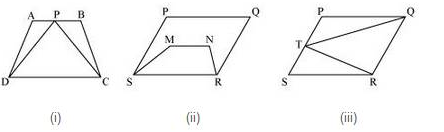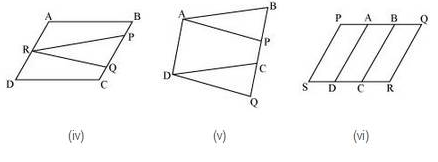# NCERT Solution for Class 9 Chapter 9 Areas of Parallelograms and Triangles Ex 9.1

In this page we have NCERT book Solutions for Class 9th Maths:Area of Parallelograms for EXERCISE 9.1 . Hope you like them and do not forget to like , social share and comment at the end of the page.

## Chapter 9 Exercise 9.1

Question 1
Which of the following figure lie on the same base and between the same paralells. In such a case, write the common  base and the two parallelsSolution:
1. Yes. Trapezium ABCD and triangle PCD are having a common base CD and these are lying between the same parallel lines AB and CD.
2. No. The parallelogram PQRS and trapezium MNRS are having a common base RS but their vertices (i.e. opposite to common base) P, Q of parallelogram and M, N of trapezium are not lying on a same line.

3. Yes. The Parallelogram PQRS and triangle TQR are having a common base QR and they are lying between the same parallel lines PS and QR.
4. No. We see that parallelogram ABCD and triangle PQR are lying between same parallel lines AD and BC but these are not having any common base.
5. Yes. We may observe that parallelogram ABCD and parallelogram APQD have a common base AD and also these are lying between same parallel lines AD and BQ.
6. No. We may observe that parallelogram PBCS and PQRS are laying on same base PS, but these are not between the same parallel lines.

## Summary

1. NCERT Solution for Class 9 Chapter 9 Areas of Parallelograms and Triangles Ex 9.1 has been prepared by Expert with utmost care. If you find any mistake.Please do provide feedback on mail. You can download the solutions as PDF in the below Link also
2. This chapter 9 has total 4 Exercise 9.1 ,9.2 ,9.3 and 9.4. This is the First exercise in the chapter.